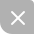# 头更更大

10月30天也就3次测试。。。为保佑进省一我还是每次测试玩都写个总结。。

boomshakalaka。。。第三题写炸。。。

# TEST 5 for NOIP（170/300）

## 鱼群分裂（100/100）

6 2

3

【样例说明】
6 分裂成 4 和 2 ，4 分裂成 1 和 3 。

【数据范围】

### MY/STD.CPP

#include<iostream>
#include<iomanip>
#include<cstdio>
#include<cstdlib>
#include<string>
#include<cstring>
#include<cmath>
#include<algorithm>
#include<queue>
using namespace std;

int dfs(int t,int k)
{
if(t%2==k%2&&t>k)
return dfs((t+k)/2,k)+dfs((t-k)/2,k);
else return 1;
}

int n,k;
int main()
{
std::ios::sync_with_stdio(false);
std::cin.tie(0);

cin >> n >> k;
if(n%2==k%2&&k==0)cout<<n<<endl;
else cout<<dfs(n,k)<<endl;
}

## 跑步

2
2 4
0 2
3 2
10 100
56 89 62 71 7 24 83 1 47 52
9 -16 34 -38 47 49 -32 17 39 -9

2/1
37/7

【数据范围】

### STD.CPP

#include<iostream>
#include<cstdio>
#include<cstring>
#include<string>
#include<algorithm>
#include<cmath>
#include<queue>
#include<vector>
#define ll long long
using namespace std;

int getint()
{
int i=0,f=1;char c;
for(c=getchar();(c<'0'||c>'9')&&c!='-';c=getchar());
if(c=='-')c=getchar(),f=-1;
for(;c>='0'&&c<='9';c=getchar())i=(i<<3)+(i<<1)+c-'0';
return i*f;
}

const int N=1e5+5,INF=1e9+5;
int T,n,L;
struct node
{
int s,v,l,r,id;
inline friend bool operator < (const node &a,const node &b)
{return a.s<b.s;}
}a[N];
struct meet
{
int x,y;
double t;
inline friend bool operator < (const meet &a,const meet &b)
{return a.t>b.t;}
};
priority_queue<meet>q;
bool del[N];

int gcd(int a,int b)
{
return !b?a:gcd(b,a%b);
}

double gettime(int x,int y)
{
if(a[x].s>a[y].s)swap(x,y);
if(a[x].v>a[y].v)return (a[y].s-a[x].s)*1.0/(a[x].v-a[y].v);
return (L-(a[y].s-a[x].s))*1.0/(a[y].v-a[x].v);
}

void W(int x,int y)
{
int dis,dv,r;
if(a[x].s>a[y].s)swap(x,y);
if(a[x].v>a[y].v)
{
dis=a[y].s-a[x].s,dv=a[x].v-a[y].v;
r=gcd(dis,dv);
dis/=r,dv/=r;
}
else
{
dis=L-(a[y].s-a[x].s),dv=a[y].v-a[x].v;
r=gcd(dis,dv);
dis/=r,dv/=r;
}
cout<<dis<<"/"<<dv<<'\n';
}

int main()
{
//freopen("run.in","r",stdin);
//freopen("run.out","w",stdout);
T=getint();
while(T--)
{
n=getint(),L=getint();

memset(del,false,sizeof(del));
while(!q.empty())q.pop();

for(int i=1;i<=n;i++)a[i].id=i,a[i].s=getint();
for(int i=1;i<=n;i++)a[i].v=getint();
sort(a+1,a+n+1);

for(int i=1;i<=n;i++)
a[i].l=i-1,a[i].r=i+1;

a.l=n,a[n].r=1;
for(int i=1;i<=n;i++)
q.push((meet){i,a[i].r,gettime(i,a[i].r)});
for(int i=1;i<=n-2;i++)
{
while(del[q.top().x]||del[q.top().y])q.pop();

int x=q.top().x,y=q.top().y,z;
q.pop();

if(a[x].id<a[y].id)swap(x,y);
del[y]=true;

if(y==a[x].l)z=a[y].l,a[x].l=z,a[z].r=x;
else z=a[y].r,a[x].r=z,a[z].l=x;

q.push((meet){x,z,gettime(x,z)});
}
while(del[q.top().x]||del[q.top().y])q.pop();
W(q.top().x,q.top().y);
}
return 0;
} 

## 拆墙（70/100）

1
4 4
-1 -1
-1 1
1 1
1 -1
1 2 1
2 3 2
3 4 1
4 1 2

1 1

【数据范围】

### MY/STD.CPP

#include<iostream>
#include<cstdio>
#include<cstdlib>
#include<cmath>
#include<ctime>
#include<algorithm>
#include<string>
#include<cstring>
#include<queue>
using namespace std;

int u,v,val,cnt=0;
int n,m;
bool visit;

struct node{
int u,v,val;
}side[100005*2];

bool comp(node a,node b){return a.val>b.val;}

int fa[100005*2];
int getfa(int x)
{
return fa[x]==x?x:fa[x]=getfa(fa[x]);//<=永远记得压缩路径啊！！！
}

int t;
int ans1;
long long sum,ans2;
int main()
{
std::ios::sync_with_stdio(false);
std::cin.tie(0);

//freopen("wall.in","r",stdin);
//freopen("wall.out","w",stdout);
cin >> t;
while(t--)
{
sum=0;ans1=0;ans2=0;cnt=0;

cin >> n >> m;
for(int i=1;i<=n;i++){cin>>u>>v;}
for(int i=1;i<=m;i++)
{
cin >> u >> v >> val;
side[++cnt].u = u;
side[cnt].v = v;
side[cnt].val = val;

sum += val;
fa[u]=u;fa[v]=v;
}

sort(side+1,side+cnt+1,comp);

for(int i=1;i<=cnt;i++)
{
if(getfa(side[i].u)!=getfa(side[i].v))
{
fa[getfa(side[i].u)] = side[i].v;
ans2 += side[i].val;
ans1 += 1;
}
}
cout << m-ans1 << " " << sum-ans2 << endl;
}
}

# NOIP初赛

2017.10.13.复习复习，明早还要考次试（曾老叫的orz），下午初赛。UP更新不错过~
11-01314
02-1811
10-14386
10-09238
10-06386
10-11489
10-19506
10-08328
10-20249
10-17644
10-16266
10-23232
10-17500
09-16274
08-29307
07-25319
09-23304
07-28322
07-28278
07-19292

### “相关推荐”对你有帮助么？

•非常没帮助
•没帮助
•一般
•有帮助
•非常有帮助被折叠的  条评论 为什么被折叠?到【灌水乐园】发言AVALON_X

¥2 ¥4 ¥6 ¥10 ¥20余额支付 (余额：-- )扫码支付获取中扫码支付点击重新获取扫码支付1.余额是钱包充值的虚拟货币，按照1:1的比例进行支付金额的抵扣。
2.余额无法直接购买下载，可以购买VIP、C币套餐、付费专栏及课程。余额充值